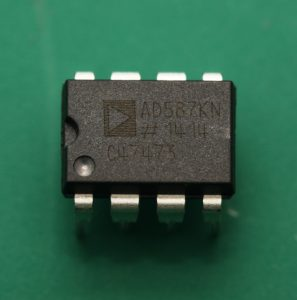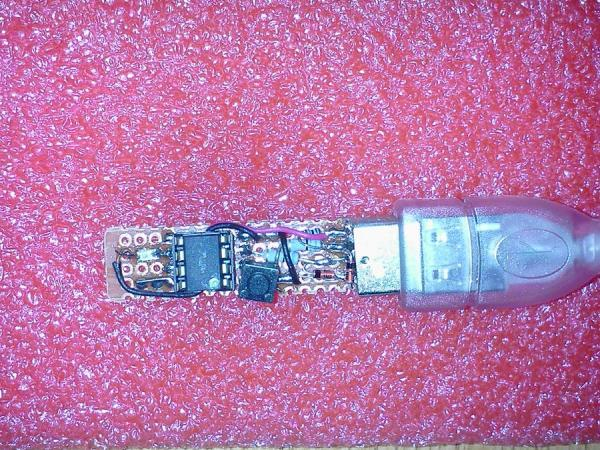# Analog Devices AD587KN 10V reference chip

Voltage references are a humble piece of hardware, their sole function is to provide a stable, known voltage. This constant, known value of voltage can then be used as a reference for ADCs and DACs as well as provide a precision current source.I recently got hold of an Analog Devices AD587KN high precision 10.000V reference chip.

This model of chip has an output value of 10.000V ± 5mV (that is, an output value of 9.995V to 10.005V) straight out of the factory. A voltage drift of 10ppm/°C at 25°C meaning that the output voltage will drift by 10μV for each 1°C the chip is exposed to. Additionally, the chip has a voltage trim input, so if you have access to a precision voltmeter, the chip’s output value can be adjusted even closer to 10.000V.

Alternatively, the chip’s output can be trimmed to a value of 10.24V. You may think that a value of 10.24V seems like a strangely familiar number. A value of 1024 is the decimal representation of 10bits, that is 2∧10 = 1024. Why would I want a voltage reference that outputs a value of 10.24V? Because it makes any ADC or DAC conversions much simpler.

For example, consider that you have a 10bit ADC and you provide it with a precise 10.000V reference voltage. Your 10bit ADC has a resolution of 10.000V/10bit = 10.000/1024 = 0.009766V/bit. Or more simply, the ADC can resolve 9.766mV (3decimal places) per bit. In any software that is manipulating data from the ADC, a scaling factor of 9.766 has to be used and it is apparent that this is a cumbersome number to deal with (let alone your software will be slowed down by having to perform floating point calculations due to the decimal point).

However, if the reference voltage is trimmed to 10.24V (a feature of the AD587), life becomes easier. Your 10bit ADC now has a resolution of 10.24V/10bit = 10.24/1024 = 0.01V/bit. Now the ADC can resolve 10mV per bit. A beautiful whole number!